#### 期刊菜单

Analysis of Flow and Heat Transfer of Maxwell-Power-Law Fluid Film on Stretching Plate
DOI: 10.12677/MP.2021.116014, PDF, HTML, XML, 下载: 125  浏览: 205  国家自然科学基金支持

Abstract: In this paper, the flow and heat transfer of Maxwell-power-law fluid film over a stretching plate are studied. Based on the rheological constitutive relationship, the velocity slip and convective heat transfer boundary conditions are modified, and the effects of magnetic field and nanoparticle on the flow are analyzed. Use appropriate similar transformations to change the governing equations into ordinary differential equations. Combining the two-parameter deformation expansion method (DPTEM), the approximate analytical solutions of the differential equation are obtained. The graph reveals the influence of various physical parameters on film thickness, velocity field and temperature field. Among them, De, M, and S have obvious effects on film thickness, while DeE and have almost no effect on film thickness. Increasing DeE and S helps fluid flow, and increasing DeE and helps to enhance the heat transfer effect.

1. 引言

2. 模型建立Table 1. Related physical parameters of base fluid and nanoparticles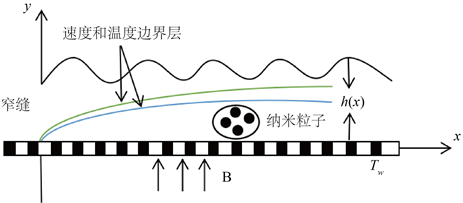Figure 1. Physical schematic

Maxwell-幂律本构方程为：

$\left(1+\lambda \frac{D}{Dt}\right)S=\mu {\stackrel{˙}{\gamma }}^{n-1}A,$ (1)

$\frac{\partial u}{\partial x}+\frac{\partial v}{\partial y}=0$ (2)

$\begin{array}{l}u\frac{\partial u}{\partial x}+v\frac{\partial u}{\partial y}+\lambda \left({u}^{2}\frac{{\partial }^{2}u}{\partial {x}^{2}}+2uv\frac{{\partial }^{2}u}{\partial x\partial y}+{v}^{2}\frac{{\partial }^{2}u}{\partial {y}^{2}}\right)\\ =\frac{{u}_{nf}}{{\rho }_{nf}}\frac{\partial }{\partial y}\left({|\frac{\partial u}{\partial y}|}^{n-1}\frac{\partial u}{\partial y}\right)-\frac{\sigma {B}^{2}}{\rho }\left[u+\lambda \left(u\frac{\partial u}{\partial x}+v\frac{\partial u}{\partial y}\right)\right]\end{array}$ (3)

$q+{\lambda }_{E}\left(\frac{\partial q}{\partial t}+V\cdot \nabla q-q\cdot \nabla V+\left(\nabla \cdot V\right)q\right)=-k{|\frac{\partial u}{\partial y}|}^{n-1}\nabla T$ (4)

$\begin{array}{l}u\frac{\partial T}{\partial x}+v\frac{\partial T}{\partial y}+{\lambda }_{E}\left(u\frac{\partial u}{\partial x}\frac{\partial T}{\partial x}+v\frac{\partial v}{\partial y}\frac{\partial T}{\partial y}+u\frac{\partial v}{\partial x}\frac{\partial T}{\partial y}+v\frac{\partial u}{\partial y}\frac{\partial T}{\partial x}+2uv\frac{{\partial }^{2}T}{\partial x\partial y}+{u}^{2}\frac{{\partial }^{2}T}{\partial {x}^{2}}+{v}^{2}\frac{{\partial }^{2}T}{\partial {y}^{2}}\right)\\ ={\alpha }_{nf}\frac{\partial }{\partial y}\left({|\frac{\partial T}{\partial y}|}^{n-1}\frac{\partial T}{\partial y}\right)\end{array}$ (5)

$u={u}_{w}-\lambda \left(\mu \frac{\partial u}{\partial x}+v\frac{\partial v}{\partial y}-u\frac{\partial {u}_{w}}{\partial x}\right)+{\lambda }_{1}{\mu }_{nf}\left({|\frac{\partial u}{\partial y}|}^{n-1}\frac{\partial u}{\partial y}\right)$ (6)

$v=-{V}_{0}$ (7)

$v=u\frac{\partial h}{\partial x},\text{}\frac{\partial u}{\partial y}=0$ (8)

${k}_{nf}{|\frac{\partial T}{\partial y}|}^{n-1}\frac{\partial T}{\partial y}={h}_{0}\left[\left(T-{T}_{w}\right)+{\lambda }_{E}\left(u\frac{\partial T}{\partial x}+v\frac{\partial T}{\partial y}-v\frac{\partial {T}_{w}}{\partial x}\right)\right]$ (9)

$\frac{\partial T}{\partial y}=0$ (10)

$u=\partial \psi /\partial y,\text{\hspace{0.17em}}v=-\partial \psi /\partial x$

$\psi ={b}^{\frac{2n-1}{n+1}}{v}_{f}^{\frac{1}{n+1}}{x}^{\frac{2n}{n+1}}f\left(\eta \right),\text{ }\text{ }\text{ }\text{ }\eta ={b}^{\frac{2-n}{n+1}}{v}_{f}^{-\frac{1}{n+1}}{x}^{\frac{1-n}{1+n}}y$

$T={T}_{0}-{T}_{ref}dbx\theta \left(\eta \right),\text{ }\text{ }\text{ }\text{ }\beta =h\left(x\right){b}^{\frac{2-n}{n+1}}{v}_{f}^{-\frac{1}{n+1}}{x}^{\frac{1-n}{1+n}}$

$\begin{array}{l}{\left({f}^{\prime }\right)}^{2}-\frac{2n}{n+1}f{f}^{″}+De\left[\frac{2\left(n-1\right)}{{\left(n+1\right)}^{2}}{\left({f}^{\prime }\right)}^{2}{f}^{″}\eta -\frac{8n}{{\left(n+1\right)}^{2}}f{f}^{\prime }{f}^{″}+{\left(\frac{2n}{n+1}\right)}^{2}{f}^{2}{f}^{‴}\right]\\ \text{ }-{\epsilon }_{1}{\left({|{f}^{″}|}^{n-1}{f}^{″}\right)}^{2}+M{f}^{\prime }-DeM\left[{\left({f}^{\prime }\right)}^{2}-\frac{2n}{1+n}f{f}^{″}\right]=0\end{array}$ (11)

$\begin{array}{l}\theta {f}^{\prime }-\frac{2n}{n+1}f{\theta }^{\prime }+D{e}_{E}\left[{\left({f}^{\prime }\right)}^{2}\theta -\frac{4n}{{\left(n+1\right)}^{2}}f{f}^{\prime }{\theta }^{\prime }-\frac{2n}{n+1}\frac{1-n}{1+n}\eta {\theta }^{\prime }{\left({f}^{\prime }\right)}^{2}-\frac{2n}{n+1}f{f}^{″}\theta +\frac{2n-{n}^{2}}{{\left(1+n\right)}^{2}}{\left({f}^{\prime }\right)}^{2}{\eta }^{2}{\theta }^{″}\right]\\ =\frac{{\epsilon }_{2}}{Pr}\left({|{\theta }^{\prime }|}^{n-1}{\theta }^{\prime }\right)\end{array}$ (12)

$\eta =0$ 时：

${f}^{\prime }\left(0\right)+De\left[{\left({f}^{\prime }\left(0\right)\right)}^{2}-{f}^{\prime }\left(0\right)-\frac{2n}{n+1}f\left(0\right){f}^{″}\left(0\right)\right]=1-\lambda {|{f}^{″}\left(0\right)|}^{n},f\left(0\right)=\frac{n+1}{2n}S$

$\theta \left(0\right)-1+D{e}_{E}\left[{f}^{\prime }\left(0\right)\cdot \theta \left(0\right)-{f}^{\prime }\left(0\right)-\frac{2n}{n+1}f\left(0\right){\theta }^{\prime }\left(0\right)\right]=\frac{{\epsilon }_{3}}{Bi}{|{\theta }^{\prime }\left(0\right)|}^{n}$ (13)

$\eta =\beta$ 时：

$f\left(\beta \right)=0,{\theta }^{\prime }\left(\beta \right)=0,{f}^{″}\left(\beta \right)=0$

$\lambda ={\lambda }_{1}{b}^{\frac{2n-1}{n+1}}{x}^{\frac{n-1}{n+1}}{v}_{f}^{-\frac{n}{n-1}},{\epsilon }_{3}=\frac{{k}_{s}+2{k}_{f}-2\varphi \left({k}_{f}-{k}_{s}\right)}{{k}_{s}+2{k}_{f}+\varphi \left({k}_{f}-{k}_{s}\right)},Bi={h}_{0}{k}_{f}^{-1}{T}_{ref}^{1-n}{d}^{1-n}{b}^{\frac{1-2n}{n+1}}{x}^{\frac{n-1}{n+1}}{v}_{f}^{\frac{n}{n+1}}$

$S={V}_{0}{b}^{\frac{1-2n}{n+1}}{v}_{f}^{-\frac{1}{n+1}}{x}_{n+1}^{1-n}$

3. DPTEM方法求解

${f}^{″}\left(0\right)=a,{\theta }^{\prime }\left(0\right)=b$ (14)

$f\left(\eta \right)={\epsilon }^{3}F\left(\xi ,\epsilon \right)+\eta +\frac{a}{2}{\eta }^{2}$ (15)

$\theta \left(\eta \right)={\epsilon }^{2}\Theta \left(\xi ,\epsilon \right)+1+b\eta$ (16)

$\eta =\epsilon \xi$ (17)

$F\left(\xi ,\epsilon \right),\Theta \left(\xi ,\epsilon \right)$$\epsilon =0$ 附近展开成幂级数形式：

$F\left(\xi ,\epsilon \right)={F}_{0}\left(\xi \right)+\underset{i=1}{\overset{\infty }{\sum }}{F}_{i}\left(\xi \right){\epsilon }^{i}$ (18)

$\Theta \left(\xi ,\epsilon \right)={\Theta }_{0}\left(\xi \right)+\underset{i=1}{\overset{\infty }{\sum }}{\Theta }_{i}\left(\xi \right){\epsilon }^{i}$ (19)

$F\left(0,\epsilon \right)=0,{F}^{\prime }\left(0,\epsilon \right)=0,{F}^{″}\left(0,\epsilon \right)=0$ (20)

$\Theta \left(0,\epsilon \right)=0,{\Theta }^{\prime }\left(0,\epsilon \right)=0$ (21)

${F}_{i}\left(0\right)=0,{F}_{i}{}^{\prime }\left(0\right)=0,{F}_{i}{}^{\prime \text{​}\prime }\left(0\right)=0,i=0,1,2,\cdots$ (22)

${\Theta }_{i}\left(0\right)=0,{{\Theta }^{\prime }}_{i}\left(0\right)=0,i=0,1,2,\cdots$ (23)

4. 结果与讨论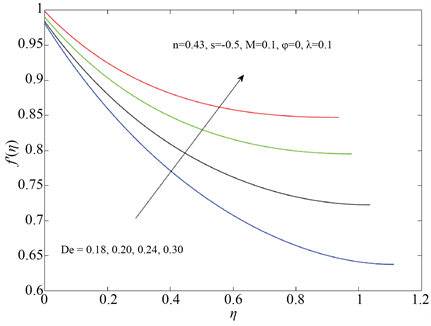Figure 2. Influence of De on the velocity field ${f}^{\prime }\left( \eta \right)$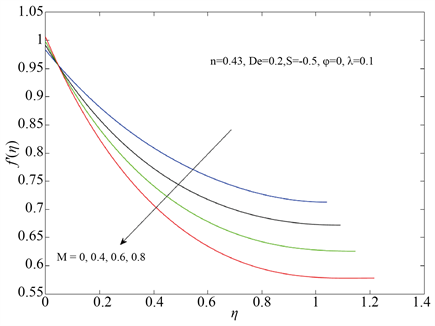Figure 3. Influence of M on the velocity field ${f}^{\prime }\left( \eta \right)$Figure 4. Influence of S on the velocity field ${f}^{\prime }\left( \eta \right)$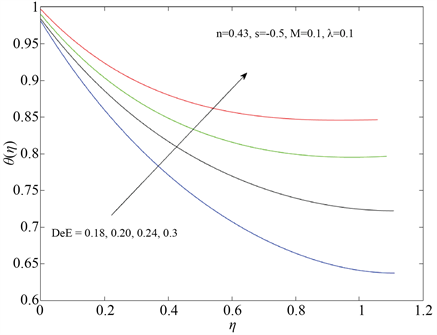Figure 5. Influence of DeE on the temperature field ${f}^{\prime }\left( \eta \right)$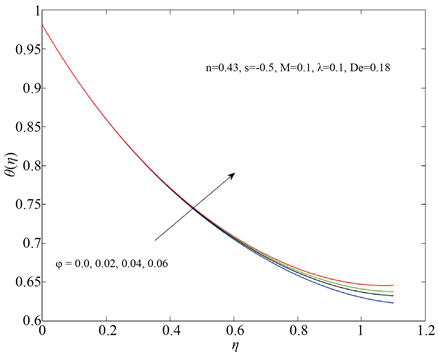Figure 6. Influence of $\varphi$ on the temperature field ${f}^{\prime }\left( \eta \right)$

5. 总结

NOTES

*通讯作者。